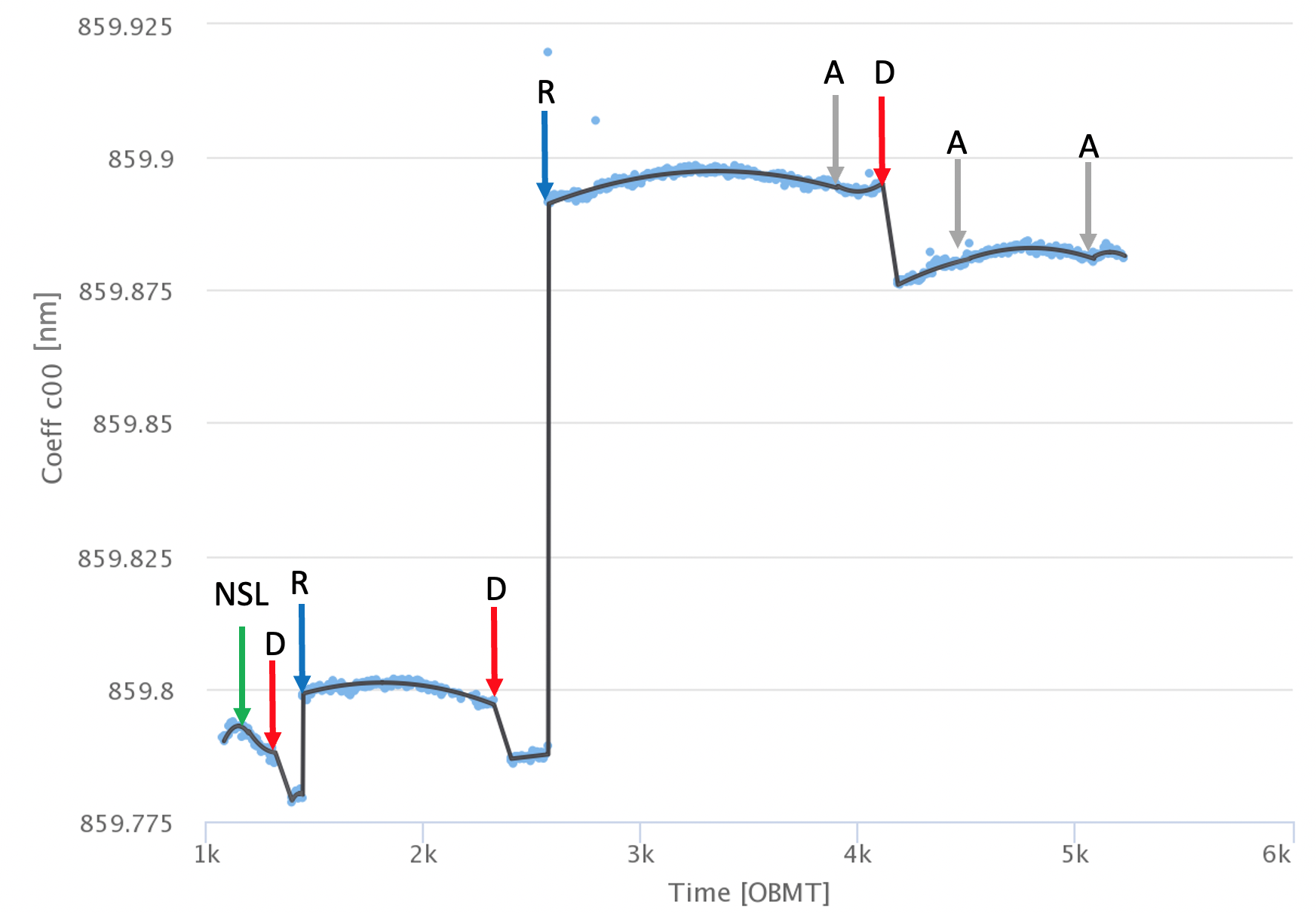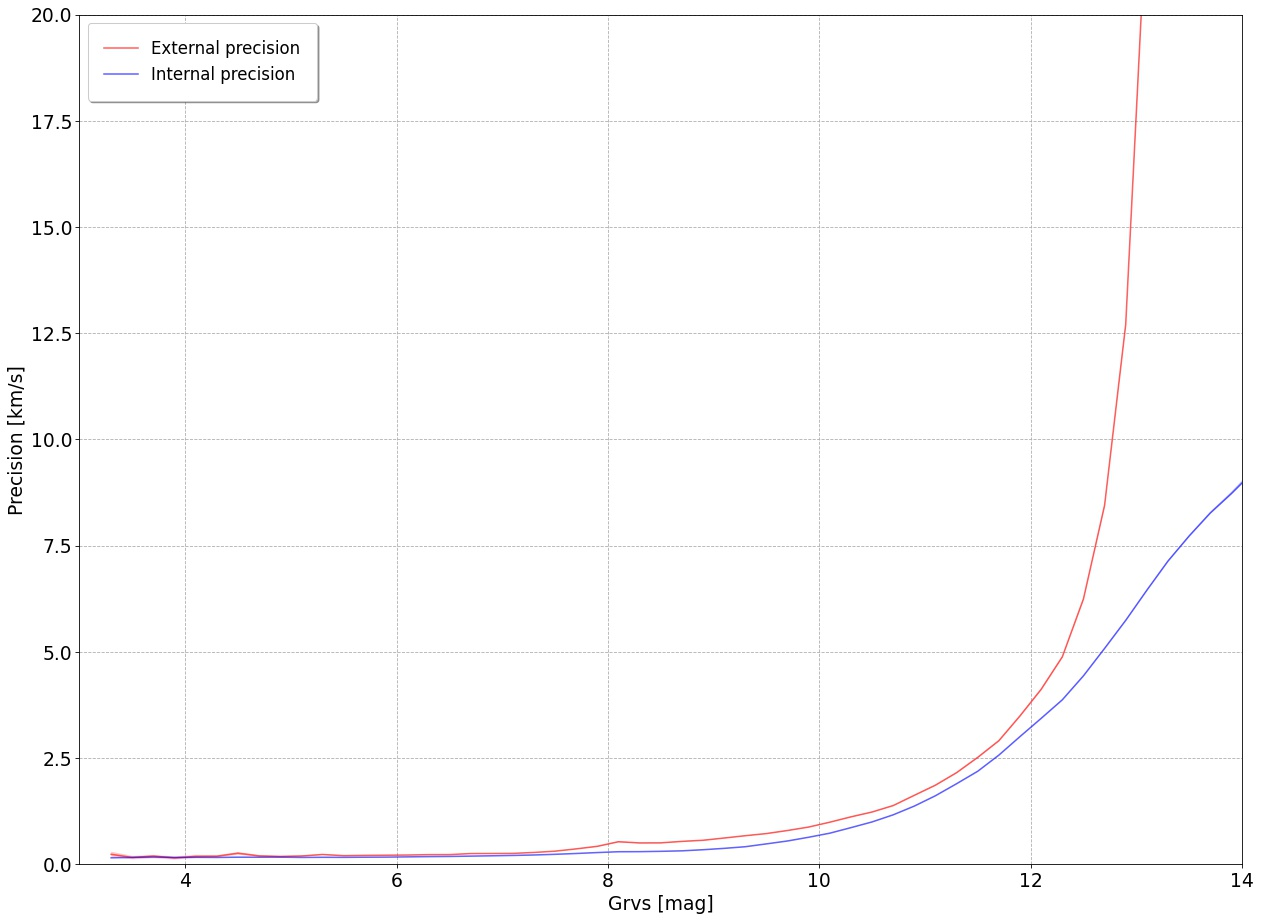# 6.5.1 Verification

The verification of the results obtained in each processing step (Section 6.4) is done automatically via the Automated Verification workflows in charge of producing diagnostic plots which permit to verify the products of each processing pipeline.

Some examples, among the many verification diagnostics, include the monitoring of the number of input and output data, of the properties of the spectra (like the window class, the blending status, etc.), of the number of invalid transits and of the invalidity reason. The electronic bias level, the total detection noise, and the barycentric velocity correction (Figure 6.10) are also monitored. In the Calibration pipeline, the number of the calibration stars and of the standard stars used in each calibration unit are monitored to verify they are in sufficient number and the trending plots of the calibration models obtained are provided for visual inspection (an example is in Figure 6.12).Figure 6.12: Wavelength calibration model: the trending function of the coefficient C00, representing the wavelength-calibration zero point, is shown for the CCD in row 5 strip 16 in FoV1 (black line). The trending functions are the final results of the wavelength calibration and are used to calibrate all the spectra acquired at any time and at any position. The time scale, on the abscissa, is expressed in OBMT (Section 1.3.1). The blue points are the values of C00 obtained at each calibration unit. The most important discontinuities are due to the re-focusing events, indicated by the blue arrows, at 1443.9 and 2574.6, and to optics decontamination, indicated by the red arrows, at OBMT 1317 and 2330.6 and 4112. Additional breakpoints are set at the transition between EPSL and NSL, at OBMT 1192.13 (green arrow), and at three discontinuities due to the OGA3 attitude at OBMT 3904, 4513.16 and 5084 (grey arrows). The breakpoints and the trending epochs are shown in Figure 6.2.

The verification of the FullExtraction pipeline includes plotting of some of the spectra that have been rejected from the data flow because of bad quality (i.e. caused by one the rejection reasons in Section 6.4.5). The total number of spectra, with $G_{\rm RVS}$ $\leq 14$ mag, that went successfully through FullExtraction is about 2 billion, about 30 % of them undergone deblending. The number of rejected spectra is approximately 855 million, the large majority (about 70 %) is rejected because requiring deblending but could not be deblended properly. Automated Verification also counts the number of cosmic rays and saturated samples detected and the number of spectra per various transit properties.

There are various automated diagnostics in charge of the verification of the STAMTA pipeline. Most of them rely on the comparison of the pipeline products with external ground-based reference data to estimate the accuracy and the external precision of the pipeline measurements as a function of grvs_mag and of the atmospheric parameters. For the radial velocities verification, the catalogues used are those listed in Table 6.3, together with the radial velocities of some open clusters (Soubiran et al. 2018a; Cantat-Gaudin et al. 2018, 2020). Despite the correction applied (see Section 6.4.8), the radial_velocities still present an overall small bias increasing with grvs_mag starting at about grvs_mag $\sim 11.5$ mag and reaching the value of about 300$\rm\,m\,s^{-1}$ at grvs_mag $\sim 14$ mag. Figure 6.13 shows the comparison of the external and internal precision for the epoch radial velocities. Some statistic information plots are also automatically produced, like the distribution of the star atmospheric parameters (Figure 6.3), or of the number of transits per star: the median number of transits of the stars having a radial_velocity is 17 and the average is 18. The good quality of the final radial velocity results is also an indirect proof of the correct behaviour of the upstream workflows.Figure 6.13: Single-transit (epoch) radial velocities external (red curve) and internal (blue curve) precision as a function of grvs_mag  for the CU6GB and the Apogee stars (see Table 6.3). The internal errors (blue curve) appear in general underestimated compared to the external precision by about 100–200m⁢s-1 for 9< grvs_mag<11.5 mag and by about 1km⁢s-1 at grvs_mag ∼12 mag. For the fainter stars the external precision rapidly decreases and the internal error is underestimated: at grvs_mag ∼13 mag, the external precision is about 20km⁢s-1 and the internal errors are underestimated by about a factor of 3. The internal error correction (Section 6.4.8) has been estimated only for the stars brighter than grvs_mag ∼12 and log Teff ≥3.5. (The multi-transit internal precision is shown in Figure 6.14). The external precision is estimated as the robust dispersion of the residuals, as described in Katz et al. (2019, Section 5.3). The single-transit radial velocities of the stars with grvs_mag >12 mag are not used to estimate the multi-transit radial_velocity. Figure by Olivier Marchal.